## Multiple Linear Regression Analysis in Excel

In a previous article, we explored Linear Regression Analysis and its application in financial analysis and modeling. You can read our Regression Analysis in Financial Modeling article to gain more insight into the statistical concepts employed in the method and where it finds application within finance.

This article will take a practical look at modeling a Multiple Regression model for the Gross Domestic Product (GDP) of a country.

Before I start, let me add a short disclaimer. I am not a statistician, and I do not claim that the selected dependent and independent variables are the right analysis choices. The article aims to show you how to run multiple Regression in Excel and interpret the output, not to teach about setting up our model assumptions and choosing the most appropriate variables.

Now that we have this out of the way and expectations are set, let’s open Excel and get started!

## Sourcing our data

We will obtain public data from Eurostat, the statistics database for the European Commission for this exercise. All the relevant source data is within the model file for your convenience, which you can download below. I have also kept the links to the source tables to explore further if you want.

The EU dataset gives us information for all member states of the union. As a massive fan of Agatha Christie’s Hercule Poirot, let’s direct our attention to Belgium.

As you can see in the table below, we have nineteen observations of our target variable (GDP), as well as our three predictor variables:

• X1 – Education Spend in mil.;
• X2 – Unemployment Rate as % of the Labor Force;
• X3 – Employee compensation in mil.Even before we run our regression model, we notice some dependencies in our data. Looking at the development over the periods, we can assume that GDP increases together with Education Spend and Employee Compensation.

## Running a Multiple Linear Regression

There are ways to calculate all the relevant statistics in Excel using formulas. But it’s much easier with the Data Analysis Tool Pack, which you can enable from the Developer Tab -> Excel Add-ins.

Look to the Data tab, and on the right, you will see the Data Analysis tool within the Analyze section.

Run it and pick Regression from all the options. Note, we use the same menu for both simple (single) and multiple linear regression models.

Now it’s time to set some ranges and settings.

The Y Range will include our dependent variable, GDP. And in the X Range, we will select all X variable columns. Please, note that this is the same as running a single linear regression, the only difference being that we choose multiple columns for X Range.

Remember that Excel requires that all X variables are in adjacent columns.

As I have selected the column Titles, it is crucial to mark the checkbox for Labels. A 95% confidence interval is appropriate in most financial analysis scenarios, so we will not change this.

You can then consider placing the data on the same sheet or a new one. A new worksheet usually works best, as the tool inserts quite a lot of data.

I will also mark all the additional options at the bottom. I rarely end up using all of them, but it’s easier to delete the ones we don’t need than rerun the whole thing.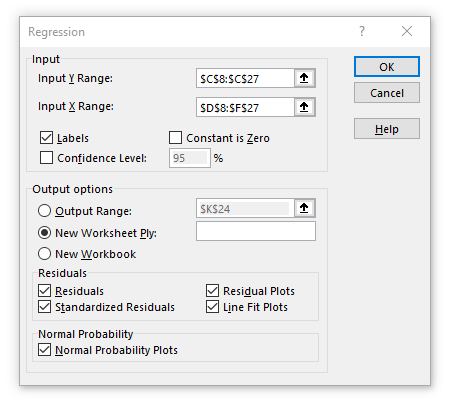## Evaluating the Regression Results

Now that we have our Summary Output from Excel let’s explore our regression model further.

The information we got out of Excel’s Data Analysis module starts with the Regression Statistics.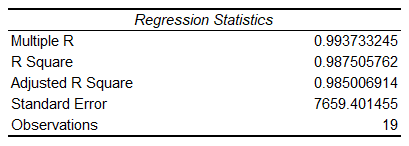R Square is the most important among those, so we can start by looking at it. Specifically, we should look at Adjusted R Square in our case, as we have more than one X variable. It gives us an idea of the overall goodness of the fit.

An adjusted R Square of 0.98 means our regression model can explain around 98% of the variation of the dependent variable Y (GDP) around the average value of the observations (the mean of our sample). In other words, 98% of the variability in ŷ (y-hat, our dependent variable predictions) is capture by our model. Such a high value would usually indicate there might be some issue with our model. We will continue with our model, but a too-high R Squared can be problematic in a real-life scenario. I suggest you read this article on Statistics by Jim, to learn why too good is not always right in terms of R Square.

The Standard Error gives us an estimate of the standard deviation of the error (residuals). Generally, if the coefficient is large compared to the standard error, it is probably statistically significant.

## Get a FREE Excel Benchmark Analysis Template

### Analysis of Variance (ANOVA)The Analysis of Variance section is something we often skip when modeling Regression. However, it can provide valuable insights, and it’s worth taking a look at. You can read more about running an ANOVA test and see an example model in our dedicated article.

This table gives us an overall test of significance on the regression parameters.

The ANOVA table’s F column gives us the overall F-test of the null hypothesis that all coefficients are equal to zero. The alternative hypothesis is that at least one of the coefficients is not equal to zero. The Significance F column shows us the p-value for the F-test. As it is lower than the significance level of 0.05 (at our chosen confidence level of 95%), we can reject the null hypothesis, that all coefficients are equal to zero. This means our regression parameters are jointly not statistically insignificant.

You can read more on Hypothesis testing in our dedicated article.

The next table gives us information about the coefficients in our Multiple Regression Model and is the most exciting part of the analysis.Here we have many details for the intercept and each of our predictors (independent variables). Let’s explore what these columns represent:

• Coefficients – these are estimates derived by the least-squares method;
• Standard error – the standard deviation of the least-squares estimates;
• T-Stat – this is the t-statistic for the null hypothesis that the coefficient is equal to zero, versus the alternative hypothesis that it is different from zero;
• The P-value for the t-test;
• Lower and Upper 95% define the confidence interval for the coefficients.

### Test of Statistical Significance

This is the test of a null hypothesis stating the coefficient has a slope of zero. We can look at the p-values for each coefficient and compare them to the significance level of 0.05.

If our p-value is less than the significance level, this means our independent variable is statistically significant for the model. Looking at our X1 to X3 predictors, we notice that only X3 Employee Compensation has a p-value of below 0.05, meaning X1 Education Spend and X2 Unemployment Rate do not seem to be statistically significant for our regression model.

As we cannot reject the null hypothesis (that the coefficients are equal to zero), we can eliminate X1 and X2 from the model. We can also confirm this because the value zero lies between the Lower and Upper confidence brackets.

We may decide to run the model without the X1 and X2 variables and evaluate whether this results in a significant drop in the adjusted R Square measure. If it doesn’t, then it’s safe to drop X1 and X2 from the regression model.

If we do that, we get the following Regression Statistics.We can see no drop in R Square, so we can safely remove X1 and X2 from our model and simplify it to a single linear regression.

### Residual Output

The residuals give information on how far the actual data points (y) deviate from the predicted data points (ŷ), based on our regression model.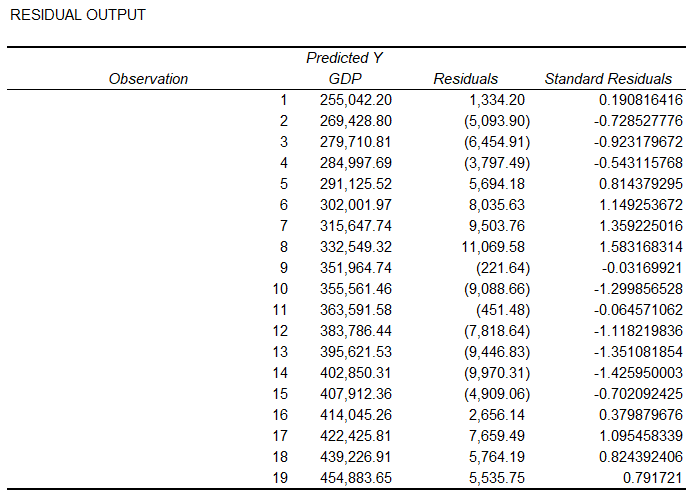### Probability Output

This table shows the observed values for the independent variable (y) and the corresponding sample percentiles. We can calculate the first percentile as (100 / 2 * Number of observations), and from there, these are calculated as the previous percentile + (100 / 2).### Residual Plots

The Multiple Regression analysis gives us one plot for each independent variable versus the residuals. We can use these plots to evaluate if our sample data fit the variance’s assumptions for linearity and homogeneity.

Homogeneity means that the plot should exhibit a random pattern and have a constant vertical spread.

Linearity requires that the residuals have a mean of zero. We can observe this visually by assessing whether the points are spread approximately equally below and above the x-axis.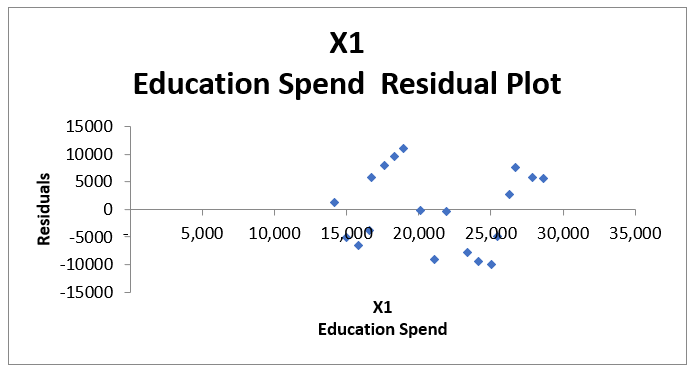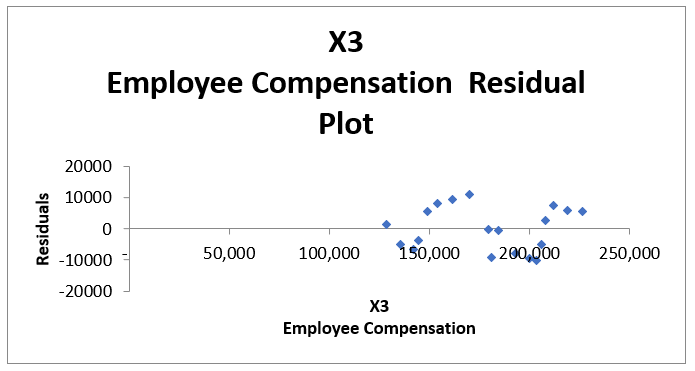### Line Fit Plots

The model provides us with one Line Fit Plot for each independent variable (predictor). This shows the predicted values (ŷ) versus the observed values (y). The closer these match, the better our model predicts the dependent variable based on the regressors.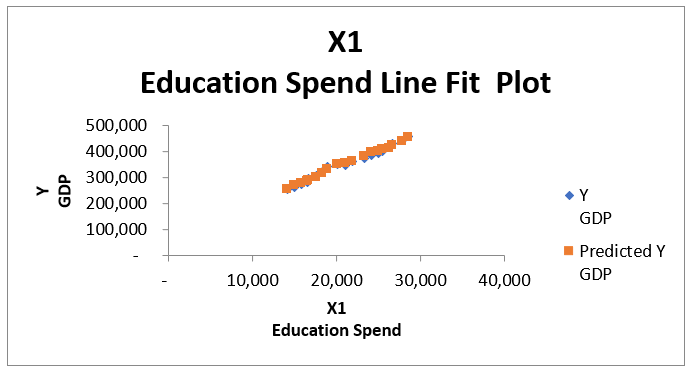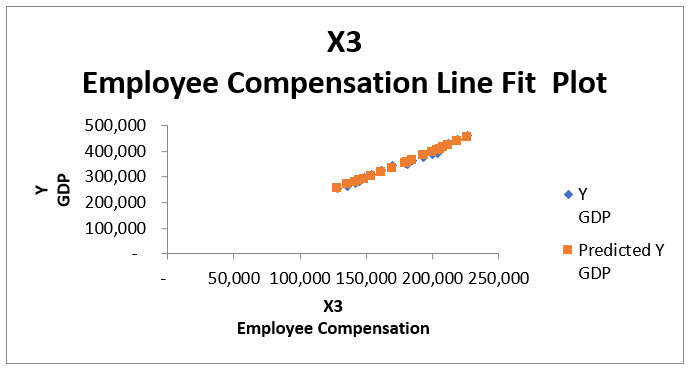### Normal Probability Plot

The Normal Probability Plot helps us determine whether the data fit a normal distribution. We can add a Trendline and evaluate if the data points follow a straight line. In our case, this is quite obvious, and we may not even add the trendline.## Excel Limitations

As Excel is not a specialized statistician software, there are some inherent limitations when running a regression model that we should be aware of:

• Columns for all regressors (independent variables) have to be adjacent;
• We can have up to 16 predictors (I can’t remember where I read that, so take it with caution);
• The regression analysis in Excel assumes the error is independent with constant variance (homoskedasticity);
• If we go the functions route, it is crucial to know that Excel functions SLOPE, INTERCEPT, and FORECAST do not work for Multiple Regression. In contrast, TREND and LINEST work the same way as with a single regression model but take values for multiple X variables.

## Conclusion

We started with three independent variables, performed a regression analysis, and identified that two predictors don’t have statistical significance for our model.

We then eliminated those to end up with a Single Linear Regression model.

Once you are satisfied with your model you can build your regression equation, as we have discussed in other articles. With this equation you can then forecast the dependent variable for the future.Where:

• y is our dependent variable;
• a is the intercept (our constant) from the regression statistics;
• b, c, and d are the coefficients for each variable;
• x1 to x3 are the independent variables (our regressors or predictors);
• ɛ is the error or residuals, which we can often exclude.

Keep in mind that this article aims to illustrate the concepts of running a Multiple Regression Analysis in Excel. It tries to explain what we should focus on when evaluating the results. Each good model starts with setting reasonable assumptions and expectations, which I am not an expert in, so I make no claims that the chosen dependent and independent variables were the right choices.

Also, don’t forget to download the Excel file below if you want to take a look at the model.## FCCA, FMVA

Hi! I am a finance professional with 10+ years of experience in audit, controlling, reporting, financial analysis and modeling. I am excited to delve deep into specifics of various industries, where I can identify the best solutions for clients I work with.

In my spare time, I am into skiing, hiking and running. I am also active on Instagram and YouTube, where I try different ways to express my creative side.

The information and views set out in this publication are those of the author(s) and do not necessarily reflect the official opinion of Magnimetrics. Neither Magnimetrics nor any person acting on their behalf may be held responsible for the use which may be made of the information contained herein. The information in this article is for educational purposes only and should not be treated as professional advice. Magnimetrics and the author of this publication accept no responsibility for any damages or losses sustained in the result of using the information presented in the publication.